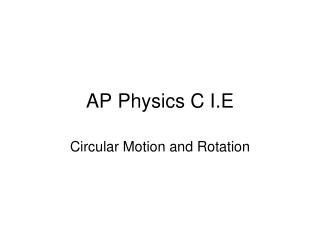DownloadDownload PresentationAP Physics C I.E

# AP Physics C I.E

Download Presentation## AP Physics C I.E

- - - - - - - - - - - - - - - - - - - - - - - - - - - E N D - - - - - - - - - - - - - - - - - - - - - - - - - - -
##### Presentation Transcript

1. AP Physics C I.E Circular Motion and Rotation

2. Centripetal force and centripetal acceleration

3. Centripetal force is the net force (sum of the forces) that keep an object moving in a circular path. It is always directed towards the center of the circle.

4. Ex. A 10.0 kg mass is attached to a string that has breaking strength of 200.0 N. If the mass is whirled in a horizontal circle of radius 80.0 cm, what maximum speed can it have? Assume the string is horizontal.

5. Ex. A roller coaster car enters the circular loop portion of the ride. If the diameter of the loop is 50 m and the total mass of the car (plus passengers) is 1200 kg, find the magnitude of the force exerted by the track on the car at a) the top of the track and b) the bottom of the track. Assume the speed of the car is 25 m/s at each location.

6. Ex. A Izzy-Dizzy-Throw-Up ride (The Gravitron) has a radius of 2.1 m and a coefficient of friction between the rider’s clothing and wall of 0.40. What minimum velocity must the Gravitron have so the rider doesn’t fall when the floor drops?

7. Ex. A stock car of mass 1600 kg travels at a constant speed of 20 m/s around a flat circular track with a radius of 190 m. What is the minimum coefficient of friction between the tires and track required for the car to make the turn without slipping?

8. FT θ Ex. The ball above makes a horizontal circular path, while the tension in the string is at an angle θ to the horizontal as shown above. Write an expression for the centripetal force on the ball.

9. Minimum speed at the top of the circle for objects making a vertical circular path • The only force at the top of the path is weight • For a roller coaster, the normal force is zero • For an object at the end of sting, tension is zero

10. Translational motion and rotational motion

11. Rotation of a body about a • Rigid body – all parts are locked together and do not change shape (a CD but not the sun; an iron bar but not a rubber hose) • Fixed axis – axis that does not move (a CD but not a bowling ball)

12. Rotational Kinematics

13. Ex. A rotating rigid body makes one complete revolution in 2.0 s. What is its average angular velocity?

14. Ex. The angular velocity of a rotating disk increases from 2.0 rad/s to 5 rad/s in 0.5 s. What is the average angular acceleration of the disk?

15. Ex. A disk of radius 20.0 cm rotates at a constant angular velocity of 6. 0 rad/s. What is the linear speed of a point on the rim of the disk?

16. Ex. The angular velocity of a rotating disk of radius 50 cm increases from 2.0 rad/s to 5.0 rad/s in 0.50 s. What is the tangential acceleration of a point on the rim of the disk during this time interval?

17. Summary for angular motion Translational Rotational Relationship Dis. s θs = rθ Vel. vωv = rω Acc. aαa = rα

18. The kinematics “Big Four” and their corresponding equations for rotational motion Linear Angular

19. Ex. An object with an initial speed of 1.0 rad/s rotates with a constant angular acceleration. Three seconds later, its angular velocity is 5.0 rad/s. Calculate the angular displacement during this time interval.

20. Ex. Starting with zero initial angular velocity, a sphere begins to spin with constant angular acceleration about an axis through its center, achieving an angular velocity of 10 rad/s when its angular displacement is 20 rad. What is the sphere’s angular acceleration?

21. First, consider torque – that which creates rotation

22. F Calculating torque – note that it is a cross product.

23. Most of the torque problems on the AP C exam involve rotating an object that is spherical or cylindrical. Therefore, the force that produces the torque is 90º to the lever arm.

24. An easy example: A student pulls down with a force of 40 N on a rope that winds around a pulley with radius of 5 cm. What is the torque on the pulley?

25. Ex. What is the net torque on the cylinder below which rotates about its center? F1 = 100 N F2 = 80 N 12 cm 8 cm

26. Now, let’s look at rotational inertia

27. Rotational inertia shows how the mass of a rotating object is distributed about the axis of rotation

28. For a point mass

29. Ex. Three beads, each of mass m, are arranged along a rod of negligible mass and length L. Find the rotational inertia when the axis of rotation is through a) the center bead and b) one of the beads on the end.

30. The parallel-axis theorem

31. For a continuous object

32. Ex. Find the rotational inertia for a uniform rod of length L and mass M rotating about its central axis.

33. Ex. Use the parallel-axis theorem to find the rotational inertia of the road about one of its ends.

34. Newton’s Second Law Translational Rotational

35. Ex. A block of mass m is hung from a pulley of radius R and mass M and allowed to fall. What is the acceleration of the block?

36. Moments of Inertia for Common Shapes

37. Kinetic Energy and Rotation

38. Rolling motion (without slipping)